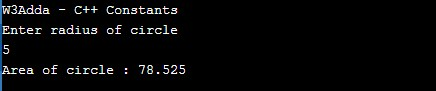# C++ Constants

In this tutorial you will learn about the C++ Constants and its application with practical example.

## C++ Constants

Constants refers to immutable values. Constants are basically literals whose values cannot be modified or changed during the execution of program. Constant are also called as Literals. Constant must be initialized when declared as values cannot be assigned it to later. In C++, constants can be of following four basic types –

## Integer Constants

An integer constant can only hold integer quantity which is a sequence of whole numbers or digits.

• Integer constant can not have a decimal point or fractional part.
• Blanks and commas are not allowed within an integer constant.
• An integer constant can be either +ve or -ve.
• The constant must lie within the range of the declared data type (including qualifiers long,short etc.).

An integer constant can be either Decimal, Hexa Decimal or Octal. A decimal integer constant consists of any combination of digits taken from the set 0 through 9. If the decimal constant contains two or more digits, the first digit can not be 0 (Zero).

Example:-

An octal integer constant is a combination of digits taken from the set 0 through 7. In octal representation first digit must be a 0(Zero), in order to identify the constant as an octal number.

Example:-

A hexadecimal integer constant must begin with either 0x or 0X. It can then be followed by any combination of digits taken from the set 0 through 9 and alphabets from A to F (either upper-case or lower-case are valid).

Example:-

## Floating Point Constants

Floating point or real constants contains a decimal point or an exponent.

• A real constant must have at least one digit each to the left and right of the decimal point.
• It can be either +ve or -ve.
• Commas and blank space are not allowed within a real constant.

A real constant can be represented in two forms: Factorial form or Exponential form. A real constant in a fractional form must have at least one digit each to the left and right of the decimal point.

Example:-

A floating-point in exponent form consists of a mantissa and an exponent.

Example:-

## Character Constants

A character constant is a single character , enclosed in single quotation marks. It can be a single alphabet, a digit or a special symbol.Maximum length of a character constant is one character.

Example:-

## String Constants

A string constant is sequence of characters enclosed in double quotes.It may contain letters, digits, special characters and blank space.

Example:-

## Defining Constants In C++

In C++, constants can be created in following two ways –

• Using #define preprocessor.
• Using const keyword.

Note:- By convention constant names are always uppercase.

## Define Constants Using #define Preprocessor

In C++, constants can be defined using #define preprocessor as following –

Syntax:-

Example:-

Output:-## Define Constants Using const Keyword

In C++, constants can be defined using const as following –

Syntax:-

Example:-

Output:-In this tutorial we have learn about the C++ Constants and its application with practical example. I hope you will like this tutorial.